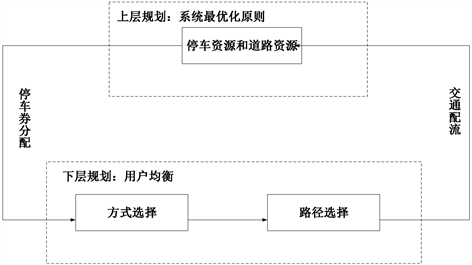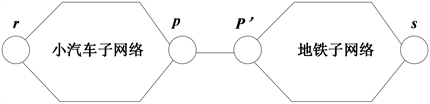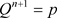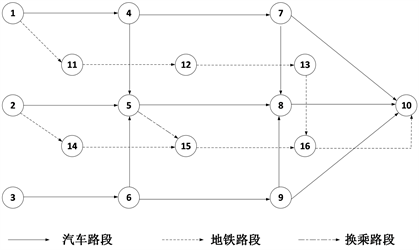# 面向大型活动的停车券优化模型Parking Permit Distribution Model for Special Events

DOI: 10.12677/MSE.2019.84039, PDF, HTML, XML, 下载: 380  浏览: 1,807

Abstract: In this paper, we study the optimal parking permit distribution schemes in different origins for special events when the destination has inadequate parking space to accommodate potential private cars. The user will choose the appropriate travel mode in terms of the parking permit distribution scheme made by the parking organizers, then make travel path decision to minimize total travel cost. Considering both parking constraint and user mode choice behavior, a bi-level programming model is proposed. The lower level programming is a mixed user mode choice equilibrium model under the condition of parking permit allocation, and the upper level programming aims to minimize the total travel cost. Finally, numerical examples are given to show that the effectiveness of the developed model, which demonstrates that parking permit distribution scheme, is efficient through increasing the use of public transport and reducing road congestion.

1. 引言

2. 模型建立Figure 1. Parking permit distribution model based on user equilibrium

2.1. 出行方式选择模型

${p}_{rs}^{ij}=\frac{{\text{e}}^{-\gamma \left({u}_{rs}^{ij}+{\upsilon }_{j}{\lambda }_{rs}-{\theta }_{i}{M}_{j}\right)}}{\underset{m\in M}{\sum }{\text{e}}^{{}^{-\gamma \left({u}_{rs}^{im}+{\upsilon }_{m}{\lambda }_{rs}-{\theta }_{i}{M}_{m}\right)}}},\text{\hspace{0.17em}}\forall i,r,s,m$ (1)

2.2. 下层多方式网络均衡模型Figure 2. Park-and-ride network structure

$\underset{f}{\mathrm{min}}z\left(f,Q\right)=\underset{l\in L}{\sum }{\int }_{0}^{{x}_{l}}{t}_{l}\left(w\right)\text{d}w+\frac{1}{\gamma }\underset{r\in R}{\sum }\underset{s\in S}{\sum }\underset{m\in M}{\sum }\left({q}_{rs}^{m}\mathrm{ln}{q}_{rs}^{m}-{q}_{rs}^{m}\right)-\underset{r\in R}{\sum }\underset{s\in S}{\sum }\underset{m\in M}{\sum }\underset{i\in I}{\sum }{\theta }_{i}{M}_{m}{q}_{rs}^{im}$ (2)

$\underset{k\in {K}_{rs}^{m}}{\sum }{f}_{ik}^{rs}={q}_{rs}^{im}\text{,}\forall r,s,i,m$ (3)

$\underset{s\in S}{\sum }\underset{m\in M}{\sum }{q}_{rs}^{im}={O}_{r}^{i}\text{,}\forall r,i$ (4)

${q}_{rs}^{a}\le {Q}_{rs}\text{,}\forall r,s$ (5)

${f}_{ik}^{rs}\ge 0,\text{}k\in {K}_{rs}^{m},\text{}\forall m,r,s,i,k$ (6)

${x}_{l}=\underset{i\in I}{\sum }\left(\underset{r\in R}{\sum }\underset{s\in S}{\sum }\underset{k\in {K}_{rs}^{a}}{\sum }{f}_{ik}^{rs}{\delta }_{lk}^{rs}+\underset{r\in R}{\sum }\underset{s\in S}{\sum }\underset{k\in {K}_{rp}^{c}}{\sum }{f}_{ik}^{rp}{\delta }_{lk}^{rp}\right),\text{}l\in {L}_{a}$ (7)

${x}_{l}=\underset{i\in I}{\sum }\left(\underset{r\in R}{\sum }\underset{s\in S}{\sum }\underset{k\in {K}_{rs}^{b}}{\sum }{f}_{ik}^{rs}{\delta }_{lk}^{rs}+\underset{r\in R}{\sum }\underset{s\in S}{\sum }\underset{k\in {K}_{p\text{'}s}^{c}}{\sum }{f}_{ik}^{{p}^{\prime }s}{\delta }_{lk}^{{p}^{\prime }s}\right),\text{}l\in {L}_{b}$ (8)

${x}_{l}=\underset{r\in R}{\sum }\underset{s\in S}{\sum }\underset{m\in M}{\sum }\underset{k\in {K}_{rs}^{m}}{\sum }\left(\underset{i\in I}{\sum }{f}_{ik}^{rs}\right)\cdot {\delta }_{lk}^{rs},\text{}\forall l\in L$ (9)

${q}_{rs}^{m}=\underset{i\in I}{\sum }{q}_{rs}^{im},\forall r,s,m$${Q}_{rs}$ 为终点s分配给起点r的停车券数量； ${t}_{l}$ 为路段通行时间，是路段流量的增函

2.3. 上层停车券分配模型

$\mathrm{min}h\left({Q}_{rs}\right)=\underset{l\in L}{\sum }{t}_{l}\left({x}_{l}\right)\cdot {x}_{l}$ (10)

$\underset{r\in R}{\sum }{Q}_{rs}\le {Q}_{s}\text{,}\forall s$ (11)

${Q}_{rs}\ge 0,\text{}\forall r,s$ (12)

${Q}_{rs}$ 是上层规划的输入变量，为终点分配给起点r的停车券数量， ${x}_{l}$ 是下层规划问题的解，是关于 ${Q}_{rs}$ 的函数。约束(11)保证终点分配给所有起点的停车券总量应不超过其自身的停车容量( ${Q}_{s}$ )限制。

3. 求解算法

$\mathrm{min}\frac{1}{2}{‖Q-\stackrel{^}{Q}‖}^{2}$ (13)

1) 利用均匀分布进行随机扰动，产生一个新的试探点 $\stackrel{^}{p}$。若 $\stackrel{^}{p}$ 满足上层约束，则取 $p=\stackrel{^}{p}$，否则令 $\stackrel{^}{Q}=\stackrel{^}{p}$，求解问题(13)得到最优解p。以p为上层决策变量取值，求解下层规划，得到最优解 $\stackrel{˜}{f}$，计算下层目标函数值 $\stackrel{˜}{h}$

2) 若 ${h}^{n}-\stackrel{˜}{h}>0$，则接受p，令${f}^{n+1}=\stackrel{˜}{f}$${h}^{n+1}=\stackrel{˜}{h}$。否则，以一定的概率决定是否接受新解：产生一个(0,1)上均匀分布的随机变量 $\sigma$，计算在当前迭代点和温度 ${T}_{k}$ 下接受点p的概率

$\rho =\mathrm{exp}\left(\frac{{h}^{n}-\stackrel{˜}{h}}{{T}_{k}}\right)$。若 $\sigma <\rho$，则令 ${Q}^{n+1}=p$${f}^{n+1}=\stackrel{˜}{f}$${h}^{n+1}=\stackrel{˜}{h}$ ；否则， ${Q}^{n+1}={Q}^{n}$${f}^{n+1}={f}^{n}$${h}^{n+1}={h}^{n}$

3) 更新 $n=n+1$，若 $n 时，继续下一次的扰动和接受过程，即执行1) 2)过程。否则，转到步骤3。

4. 算例分析Figure 3. Network of numerical example

4.1. 参数设置

${t}_{l}\left({x}_{l}\right)={t}_{l}^{0}\left[1+\alpha {\left(\frac{{x}_{l}}{{C}_{l}}\right)}^{\beta }\right],\forall l\in L$ (14)

$\gamma$ 取值为1。

4.2. 算例结果和效率分析Table 2. Traffic equilibrium results with optimal parking permit distributionFigure 4. Traffic flow of different travel mode

5. 结论

  吕能超. 大型活动动态交通组织方法建模研究[D]: [博士学位论文]. 武汉: 武汉理工大学, 2010.  Qian, Z., Xiao, F. and Zhang, H.M. (2012) Managing Morning Commute Traffic with Parking. Transportation Research Part B, 46, 894-916. https://doi.org/10.1016/j.trb.2012.01.011  Zhang, X., Yang, H. and Huang, H.J. (2011) Improving Travel Efficiency by Parking Permits Distribution and Trading. Transportation Research Part B Methodological, 45, 1018-1034. https://doi.org/10.1016/j.trb.2011.05.003  Liu, W., Yang, H., Yin, Y., et al. (2014) A Novel Permit Scheme for Managing Parking Competition and Bottleneck Congestion. Transportation Research Part C, 44, 265-281. https://doi.org/10.1016/j.trc.2014.04.005  Sarasua, W.A., Chowdhury, M.A. and Malisetty, P. (2006) Optimal Parking Reallocation for Special Events: Geographic Information System Analysis. Transportation Research Board Meeting, 22-26 January 2006, Washington DC, 17 p.  殷焕焕, 关宏志, 秦焕美, 等. 基于非集计模型的居民出行方式选择行为研究[J]. 武汉理工大学学报(交通科学与工程版), 2010, 34(5): 1000-1003.  Daskin, M.S. (1985) Urban Transportation Networks: Equilibrium Analysis with Mathematical Programming Methods by Yosef Sheffi. Transportation Science, 19, 463-466. https://doi.org/10.1287/trsc.19.4.463  査伟雄, 孙敬. 基于模拟退火算法的危险货物道路运输路径优化双层规划模型[J]. 公路交通科技, 2012, 29(4): 101-106.  黄海军, 李志纯. 组合出行方式下的混合均衡分配模型及求解算法[J]. 系统科学与数学, 2006, 26(3):352-361.  温旭丽, 杨涛, 马香. 基于轨道交通的停车换乘系统网络均衡模型研究[J]. 公路交通科技(应用技术版), 2016, 12(8): 261-264.  Zhang, X., Zhang, H.M., Huang, H.J., et al. (2011) Competitive, Cooperative and Stackelberg Congestion Pricing for Multiple Regions in Transportation Networks. Transportmetrica, 7, 297-320. https://doi.org/10.1080/18128602.2010.502547